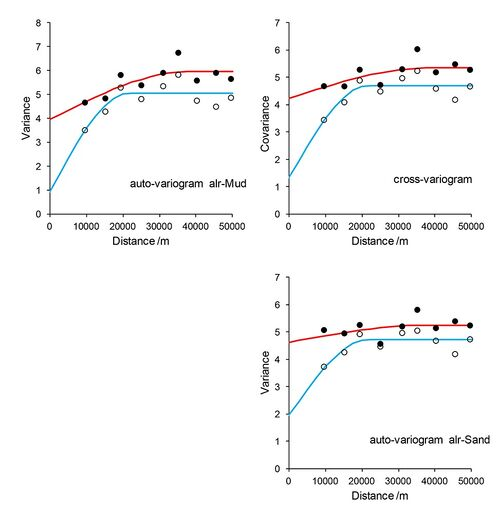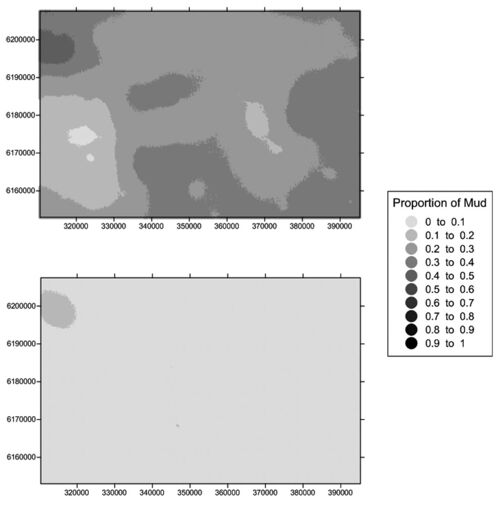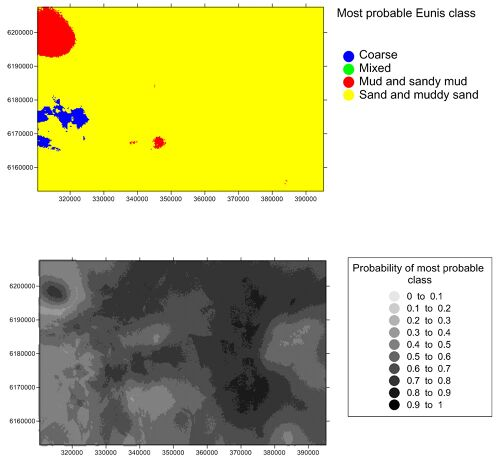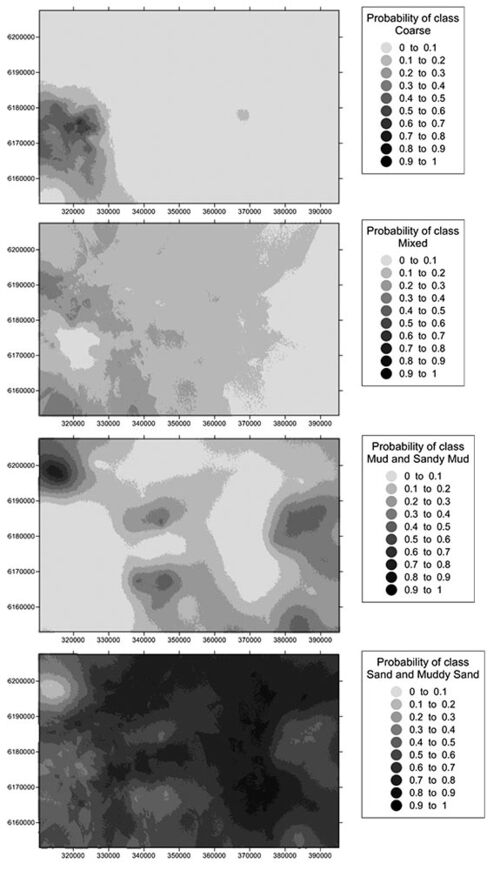# OR/14/015 Swallow Sand

 Lark R M. 2014. Mapping seabed sediments of the Swallow Sand and South-west Deeps (West) MCZs. British Geological Survey Internal Report, OR/14/015.

## Data

The data used are particle size analyses from the verification survey carried out by Cefas in 2012 (Ware, 2012). The data were collected by 0.1 m2 Hamon grab from 103 locations on a pre-planned survey grid. Exploratory analysis of the data showed that sample stations 465 and 467 have duplicate coordinates. Because such duplicated data cannot be used in the methodology of Lark et al. (2012) these two observations were excluded, leaving 101 observations. This would generally be regarded as sufficient for spatial modelling (Webster and Oliver, 1992). All analyses were therefore completed on this data set, since it is preferable to use data which are collected on a common support (the size and shape of the sample volume) and produced by a consistent analytical methodology.

The data provided are percent by mass of gravel (particles diameter >2 mm), mud (particles diameter <0.063 mm) and sand (particles 2 mm > diameter >0.063 mm).

### Exploratory data analysis and transformation

Exploratory statistics of the 101 particle size data are shown in Table 1 below. Note that two zero values were recorded for gravel content. As explained by Lark et al. (2012) zero values cannot be subject to the additive log-ratio transformation that is required for compositional variates such as particle size data. We therefore imputed a small value (0.005%) for all zero observations then renormalized the values to sum to 100 for each observation. This is the same procedure used by Lark et al. (2012).

The particle size data were then subject to an additive log-ratio (alr) transformation. This replaces a n-variate composition with n–1 transformed values, the natural logarithm of the ratio of two of the components of the composition to the third. In this case we used the gravel content as the denominator of the log-ratio so our two variables are alrM and alrS where

alrM = loge (mud content/gravel content) (1)
alrS = loge (mud content/gravel content) (2)

where mud content, gravel content and sand content are all percent by mass. Note that the choice of component to form the denominator of the log-ratio does not affect the outcome of spatial prediction by compositional cokriging (Pawlowsky-Glahn and Olea, 2004). We decided to use gravel as the denominator because it gave log-ratios of similar variability. Table 2 shows summary statistics of the alr-transformed data.

 Gravel/% Mud/% Sand/% Mean 7.84 12.71 79.45 Minimum 0.00 1.74 35.27 Maximum 60.03 40.30 97.94 StandardDeviation 13.25 6.89 13.09
 alr-mud alr-sand Mean 2.28 4.25 Minimum 2.70 4.53 Standarddeviation 2.53 2.42 Skewness –0.097 –0.147

## Spatial analysis

Auto-variograms and cross-variograms of the alr-transformed data were estimated from the data. The same method-of-moments estimator (MoM) was used as described by Lark et al. (2012). However, a different robust estimator was used to the previous study, the minimum volume ellipsoid (MVE) estimator of Rousseeuw (1984) adapted for estimation of auto- and cross-variograms by Lark (2003).

Lark (2003) compared the MVE estimator with an alternative due to Ma and Genton (2001) and found it to be the more robust. However, Lark et al. (2012) did not use MVE because it was too computationally demanding for use on their large data set. In the context of the current study, however, it could be used.

The linear model of coregionalization (LMCR) was fitted to the MoM and MVE estimates of the auto- and cross-variograms by weighted least squares, as described by Lark et al. (2012). The fitted models are shown in Figure 1 below.Figure 1    Estimated variograms and cross-variograms with fitted linear model of coregionalizations for alr-mud and alr-sand for Swallow Sand. Solid symbols are method of moment estimates with fitted model in red. Open symbols are mimimum volume ellipsoid estimates with fitted model in blue.

The LMCRs were then compared by cross-validation, in which each observation was predicted by ordinary kriging from all remaining data. This was described in detail by Lark et al. (2012). The key diagnostic is the standardized squared prediction error, q (x), which is the square of the difference between the cross-validation prediction and the known value at location x, standardized by the ordinary kriging variance. If the kriging variance is, on average, an appropriate descriptor of the prediction uncertainty, then the mean of q (x) over all locations should be close to 1.0. As Lark et al. (2012) explain the median is preferred as a diagnostic because of its robustness, this should take a value close to 0.455. Larger values suggest that the kriging variance is under-estimated and smaller that it is overestimated.

 Method of Moments Minimum Volume Ellipsoid EstimatorVariable alr-mud alr-sand alr-mud alr-sand Mean of θ (x) 0.99 0.98 2.35 1.76 Median of θ (x) 0.53 0.44 1.03 0.78

The cross-validation results for the auto-variograms based on MoM estimates give results very close to those expected for the correct model. This suggests that the data are not affected by outlying values. Consistently with this the auto-variograms based on the MVE estimates seem markedly to underestimate the kriging variance. Lark (2003) found that robust estimators could be biased in the absence of outliers. On this basis the LMCR based on the MoM estimates can be used in further work. Its parameters are given in Table 4 below.

 Component Spatial correlationmodel type Distance parameter ofspatial model/metres Variance or Covariance Component Auto-variogram Cross-variogram alr-mud alr-sand 1 Nugget N.A. 3.95 4.62 4.22 2 Spherical 38500 2.00 0.63 1.12

## Spatial predictions

Lark et al. (2012) describe the cokriging procedure used to obtain conditional expectations of the transformed variables and covariance matrices for these at target points. This procedure was undertaken to form predictions at nodes of a 250-m grid. The simulation method used by Lark et al. (2012) was then used to generate 5000 independent realizations from the joint prediction distribution at each node. For each realization a back-transformation was undertaken to give values of gravel, mud and sand. Over all realizations the mean value of gravel, mud and sand were computed as the conditional expectation of these variables, and the 0.025 and 0.975 quantiles of the realizations were computed as confidence intervals for the predictions. It should be noted that these predictions and confidence intervals should be considered for each variable in turn. Further work is required on how conditional expectations and their uncertainty for compositional variables are most effectively expressed and communicated.

For each realization, the EUNIS level 3 sediment texture classes (Long, 2006) were identified. At each grid node the proportion of realizations that occurred in each class is an estimate of the conditional probability of finding that class at the location. One may report the probability for each class, one may also report the class of maximum probability. The uncertainty attached to treating a site as if the class of maximum probability were the true class there can be evaluated by examining that maximum probability value which may range from just over 1/k (where k is the number of classes) to 1.0.

The results of this analysis are held in two files.

SS_Predictions.dat is an ASCII format file. Each row corresponds to a node on the 250-m grid. The variables in each column of the file are tabulated below.

 Column Content 1 x-coordinate, UTM29N 2 y-coordinate, UTM29N 3 Estimated conditional expectation of gravel content (proportion) 4 Estimated conditional expectation of sand content (proportion) 5 Estimated conditional expectation of mud content (proportion) 6 0.025 quantile of gravel content (proportion) 7 0.975 quantile of gravel content (proportion) 8 Width of the 95% confidence interval of sand content (proportion) 9 0.025 quantile of sand content (proportion) 10 0.975 quantile of sand content (proportion) 11 Width of the 95% confidence interval of sand content (proportion) 12 0.025 quantile of mud content (proportion) 13 0.975 quantile of mud content (proportion) 14 Width of the 95% confidence interval of mud content (proportion)

Figure 2 below shows the conditional expectation of gravel, sand and mud across the Swallow Sand MCZ, and Figure 3 shows the 0.025 and 0.975 quantile which define a 95% confidence interval for mud content.Figure 2    Conditional Expectation of gravel, sand and mud content (proportions) across the Swallow Sand MCZ based on multiple realizations of the prediction distribution.Figure 3    Upper (top) and lower (bottom) bounds of the 95% confidence interval for mud content (proportion) across the Swallow Sand MCZ based on multiple realizations of the prediction distribution.

SS_classes.dat dat is an ASCII format file. Each row corresponds to a node on the 250-m grid. The variables in each column of the file are tabulated below.

 Column Content 1 x-coordinate, UTM29N 2 y-coordinate, UTM29N 3 Most probable EUNIS class:1 Coarse2 Mixed3 Mud and sandy mud4 Sand and muddy sand 4 Probability of most probable class 5 Probability of class Coarse 6 Probability of class Mixed 7 Probability of class Mud and sandy mud 8 Probability of class Mud and muddy sand 9 Entropy of the class probabilities (-1 times the expected value of the log probability over the distribution)

Figure 4 below shows the most probable EUNIS class across the Swallow Sand MCZ, and the probability of the most probable class. Figure 5 shows the probability of each class.Figure 4    Most probable EUNIS class (top) across the Swallow Sand MCZ and probability of the most probable class (bottom).Figure 5    Probability of finding each EUNIS class (top) across the Swallow Sand MCZ.

Note that, while the class Sand and Muddy Sand is delineated as most probable across most of the MCZ, there is uncertainty attached to this, particularly in the south-western and central eastern parts of the zone. This reflects the subtle transitions of sediment texture shown in Figure 2. It also reflects the importance of short-range variability of sediment texture in this MCZ. The short-range variability of sediment texture is large, shown by the relative magnitude of the nugget component and spatially correlated component of the LMCR (see variance components in Table 4). This substantial short-range variability means that there is inevitably a good deal of uncertainty attached to spatial predictions made by kriging from what is, over most of the MCZ, a relatively coarse grid.